• ## python置信区间

千次阅读 2019-10-11 22:37:00
置信区间是指由 样本统计量 所构造的总体参数的估计区间。 这句话也就是说 ( 这里统计量一般指均值 ) 利用样本均值来估计总体均值的可靠程度, 这个"可靠程度"用"置信区间"来表示, 置信区间(是一个系数)取值范围为: ...
置信区间是指由  样本统计量  所构造的总体参数的估计区间。
这句话也就是说  ( 这里统计量一般指均值 )  利用样本均值来估计总体均值的可靠程度, 这个"可靠程度"用"置信区间"来表示, 置信区间(是一个系数)取值范围为: 0<置信区间<1; 这个置信区间就是用来描述真实的均值发生在某范围的概率
当样本量越大, 则越可靠.
置信区间计算公式:
ci = mean±stdN(ppf)(  (1-α)/2  )
公式参数说明:
ci: 表示置信区间
mean: 表示样本均值
std: 表示样本标准差
N(ppf): 表示正态分布的百分点函数
α : 是显著性水平
α的取值跟样本量有关

样本量个数
α取值

0~99
0.1

100~500
0.05

501~1000
0.01

约2000
0.001

其中;百分点函数ppf是累积分布函数cdf的反函数
常用的几个置信区间的计算可以不用上述公式,而使用更简单的公式
置信水平是指特定个体对待特定命题真实性相信的程度
通常使用 90%，95% 和 99% 作为置信区间的置信水平。这三个置信水平的临界值分别为 1.64，1.98 和 2.32。
se是样本标准误, n是样本个数
se = mean/pow(n,0.5)
置信区间算法:
ci = mean-se1.64      置信水平为0.9
ci = mean-se1.98      置信水平为0.95
ci = mean-se*2.32      置信水平为0.99
举例来说，如果在一次大选中某人的支持率为55%，而置信水平(也称为可信区间)0.95以上的置信区间是(50%,60%)，那么他的真实支持率有百分之九十五的机率落在百分之五十和百分之六十之间，因此他的真实支持率不足一半的可能性小于百分之2.5。 如例子中一样，置信水平一般用百分比表示，因此置信水平0.95上的置信空间也可以表达为:95%置信区间。置信区间的两端被称为置信极限。对一个给定情形的估计来说，显著性水平越高，所对应的置信区间就会越大。
但是; 重点来了, 在python里可以直接调用函数求出置信区间
置信水平 = 可信区间 = 可信度 ;他们是同一个说法(其中可信度是为了方便理解,在案例中我用于替代置信水平的)
置信区间宽度 = 置信区间上极限 - 置信区间下极限
结论是:置信区间宽度越小,预测模型越精确;但是置信区间宽度小了,可信度也就越小了
"""  置信区间  """

df = len(data) - 1
alpha=    # 是设定的可信区间, 可以理解为可信度; 数值是百分数,取值范围(0,1)
ci = stats.t.interval(alpha, df, loc=np.mean(data), scale=stats.sem(data)) # ci是置信区间

案例: 对给出的样本数据分析(所用文件是csv格式的,无法导入)
import os
import pandas as pd
import matplotlib
import matplotlib.pyplot as plt
from scipy import stats
import numpy as np

os.chdir(r"D:\python金融")
data = pd.read_csv("house_price_gr.csv",encoding='gbk') # 文件有中文,encoding= 'gbk'

matplotlib.rcParams['axes.unicode_minus']=False#解决保存图像时负号'-'显示为方块的问题
plt.rcParams['font.sans-serif'] = ['SimHei'] # 指定默认字体

# print(data.shape) # 查看样本数量

# 绘制直方图 plt.hist(样本数据,bins=柱子个数) ; 默认x轴是样本数值, y轴是样本数值的个数
# plt.hist(data.rate,bins=40)
# plt.show()

# 查看均值,标准差,百分位数等
print(data.rate.describe())

alpha = 0.99
alpha_list = [] # 可信度列表
ci_width_list = [] # 置信区间宽度列表
ci_upper_limit = [] # 置信区间上极限
ci_lower_limit = [] # 置信区间下极限
for i in range(20):
print("alpha={}".format(alpha))
# 均值的99%可信区间的置信区间
"""  置信区间  """
df = len(data.rate) - 1
ci = stats.t.interval(alpha, df, loc=np.mean(data.rate), scale=stats.sem(data.rate))
# ci = stats.t.interval(alpha=0.99,df=len(data.rate)-1,loc=np.mean(data.rate),scale=stats.sem(data.rate))

# 算出置信区间的宽度
ci_element = ci

ci_lower_limit.append(ci_element)
ci_upper_limit.append(ci_element)
# ci_element.append(ci)
ci_width = ci_element - ci_element # 定义置信区间宽度
ci_width_list.append(ci_width)
print(ci_width)  # 输出置信区间宽度

alpha_list.append(alpha) # 添加到列表中
alpha = alpha - 0.05
print("置信区间={}".format(ci))
print("  "*100)

print(ci_width_list)
print(alpha_list)
x=alpha_list # 设置可信度为x轴
# y=ci_width_list

plt.plot(x,ci_lower_limit,linestyle="dashdot",label="置信区间下极限")
plt.plot(x,ci_width_list,linestyle="dashdot",label = "置信区间宽度")
plt.plot(x,ci_upper_limit,linestyle="dashdot",label= "置信区间上极限")
plt.xlabel("可信度/百分数") # 可信度指的是可信区间, 为了方面理解,用可信度代替可信区间
plt.ylabel("置信区间/单位为变量的单位")
plt.title("可信度--置信区间宽度 图")
plt.legend() # 显示标签
"""
可信度与置信区间图说明:
例如:可信度=0.90,置信区间宽度=0.05, 置信区间上限=0.07,置信区间下限=0.02
则说明:发生在区间(0.02,0.07)这个范围内的可能性为0.90

通过图像得出: 置信区间宽度越越小,预测模型越精确;但是置信区间宽度小了,可信度也就越小了
"""
plt.show()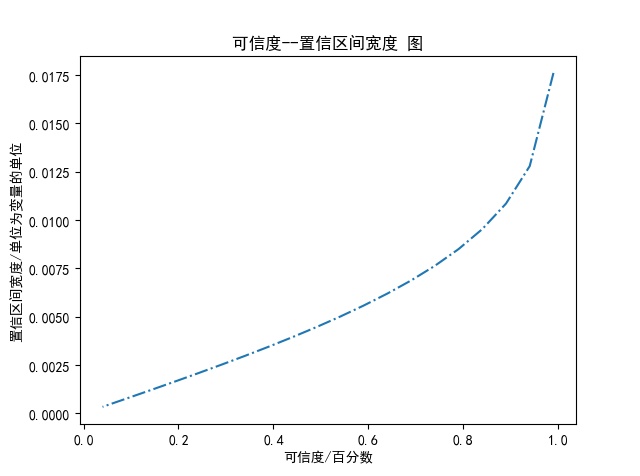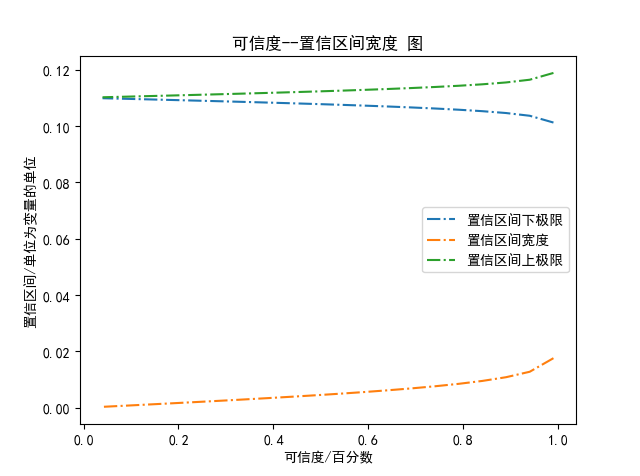通过图可以得出上述结论:
:置信区间宽度越小,预测模型越精确;但是置信区间宽度小了,可信度也就越小了
所有为了得到较高的可信度, 就必须得扩大置信区间宽度; 一般取可信度=0.95来计算


展开全文置信水平
• python 置信区间Confidence Interval (CI) is essential in statistics and very important for data scientists. In this article, I will explain it thoroughly with necessary formulas and also demonstrate ...
python 置信区间Confidence Interval (CI) is essential in statistics and very important for data scientists. In this article, I will explain it thoroughly with necessary formulas and also demonstrate how to calculate it using python. 置信区间(CI)在统计中至关重要，对数据科学家而言非常重要。 在本文中，我将用必要的公式彻底解释它，并演示如何使用python计算它。
置信区间 (Confidence Interval)
As it sounds, the confidence interval is a range of values. In the ideal condition, it should contain the best estimate of a statistical parameter. It is expressed as a percentage. 95% confidence interval is the most common. You can use other values like 97%, 90%, 75%, or even 99% confidence interval if your research demands. Let’s understand it by an example: 听起来，置信区间是一个值范围。 在理想条件下，它应包含统计参数的最佳估计。 用百分比表示。 95％的置信区间是最常见的。 如果您的研究需要，可以使用其他值，例如97％，90％，75％甚至99％置信区间。 让我们通过一个例子来理解它：
Here is a statement: 这是一条声明：
“In a sample of 659 parents with toddlers, about 85%, stated they use a car seat for all travel with their toddler. From these results, a 95% confidence interval was provided, going from about 82.3% up to 87.7%.” “在659位有学步的父母的样本中，大约85％的受访者表示，他们在学步时都使用汽车安全座椅。 根据这些结果，提供了95％的置信区间，从大约82.3％上升到87.7％。”
This statement means, we are 95% certain that the population proportion who use a car seat for all travel with their toddler will fall between 82.3% and 87.7%. If we take a different sample or a subsample of these 659 people, 95% of the time, the percentage of the population who use a car seat in all travel with their toddlers will be in between 82.3% and 87.7%. 该声明意味着，我们有95％的把握确定，带小孩旅行的所有年龄段的孩子所占的比例将介于82.3％和87.7％之间。 如果我们对这659名人群进行不同的抽样或子抽样，则有95％的时间，在与孩子一起旅行的所有时间中使用汽车安全座椅的人口比例将在82.3％至87.7％之间。
Remember, 95% confidence interval does not mean 95% probability 请记住，95％的置信区间并不意味着95％的概率 The reason confidence interval is so popular and useful is, we cannot take data from all populations. Like the example above, we could not get the information from all the parents with toddlers. We had to calculate the result from 659 parents. From that result, we tried to get an estimate of the overall population. So, it is reasonable to consider a margin of error and take a range. That’s why we take a confidence interval which is a range. 置信区间如此受欢迎且有用的原因是，我们无法从所有人群中获取数据。 像上面的示例一样，我们无法从所有有学步的父母那里获得信息。 我们必须计算659位父母的结果。 根据该结果，我们试图获得总体人口的估计值。 因此，考虑误差幅度并取一个范围是合理的。 这就是为什么我们将置信区间设为一个范围。
We want a simple random sample and a normal distribution to construct a confidence interval. But if the sample size is large enough (30 or more) normal distribution is not necessary. 我们想要一个简单的随机样本和一个正态分布来构造一个置信区间。 但是，如果样本大小足够大(30个或更多)，则不需要正态分布。 GIPHYGIPHY  如何计算置信区间 (How to Calculate the Confidence Interval)
The calculation of the confidence interval involves the best estimate which is obtained by the sample and a margin of error. So, we take the best estimate and add a margin of error to it. Here is the formula for the confidence interval and the margin of error: 置信区间的计算涉及通过样本获得的最佳估计值和误差范围。 因此，我们采用最佳估计，并在其中增加误差范围。 这是置信区间和误差范围的公式：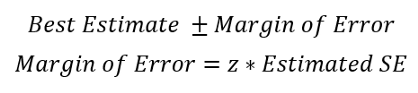Here, SE is the standard error. 在此，SE是标准误差 。
Normally, CI is calculated for two statistical parameters: the proportion and the mean. 通常，CI是针对两个统计参数计算的：比例和平均值。 Combining these two formulas above, we can elaborate the formula for CI as follows: 结合以上两个公式，我们可以将CI的公式阐述如下：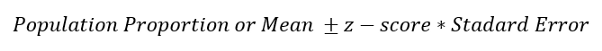Population proportion or the mean is calculated from the sample. In the example of “the parents with toddlers”, the best estimate or the population proportion of parents that uses car seats in all travel with their toddlers is 85%. So, the best estimate (population proportion) is 85. z-score is fixed for the confidence level (CL). 人口比例或平均值是从样本中计算得出的。 在“有小孩的父母”的示例中，最佳估计或在与小孩一起旅行的所有时间中使用汽车安全座椅的父母的人口比例为85％。 因此，最佳估计值(人口比例)为85。z值固定为置信度(CL)。
A z-score for a 95% confidence interval for a large enough sample size(30 or more) is 1.96. 95％置信区间的z评分对于足够大的样本量(30个或更多)为1.96。 Here are the z-scores for some commonly used confidence levels: 以下是一些常用置信度水平的z得分：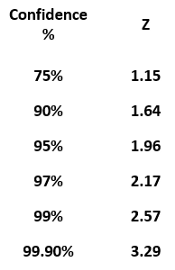The method to calculate the standard error is different for population proportion and mean. The formula to calculate standard error of population proportion is: 总体比例和均值的标准误差的计算方法不同。 计算人口比例标准误的公式为：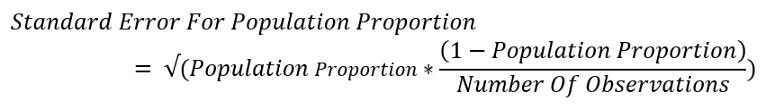The formula to calculate the standard error of the sample mean is: 计算样本平均值的标准误的公式为：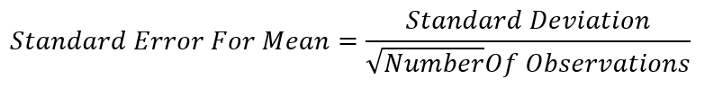As per the statement, the population proportion that uses a car seat for all travel with their toddlers is 85%. So, this is our best estimate. We need to add the margin of error to it. To calculate the margin of error we need the z-score and the standard error. I am going to calculate a 95% CI. The z-score should be 1.96 and I already mentioned the formula of standard error for the population proportion. Plugging in all the values: 根据声明，在所有带小孩的旅行中使用汽车安全座椅的人口比例为85％。 因此，这是我们的最佳估计。 我们需要添加误差范围。 要计算误差幅度，我们需要z分数和标准误差。 我要计算95％CI。 z分数应为1.96，我已经提到了人口比例的标准误公式。 插入所有值：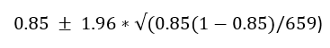The confidence interval is 82.3% and 87.7% as we saw in the statement before. 正如我们在之前的声明中所看到的，置信区间为82.3％和87.7％。
演示地址GIPHYGIPHY  Python中的置信区间 (Confidence interval in Python)
I am assuming that you are already a python user. But even if you are not a python user you should be able to get the concept of the calculation and use your own tools to calculate the same. The tools I used for this exercise are: 我假设您已经是python用户。 但是，即使您不是python用户，您也应该能够获得计算的概念，并使用自己的工具进行计算。 我用于此练习的工具是：
Numpy Library 脾气暴躁的图书馆 Pandas Library 熊猫图书馆 Statsmodels Library 统计模型库 Jupyter Notebook environment. Jupyter Notebook环境。 If you install an anaconda package, you will get a Jupyter Notebook and the other tools as well. There are some good youtube videos to demonstrate how to install anaconda package if you do not have that already. 如果安装了Python包装，则还将获得Jupyter Notebook和其他工具。 有一些不错的youtube视频，以演示如何安装anaconda软件包(如果尚未安装的话)。
CI代表Python中的人口比例 (CI for the population Proportion in Python)
I am going to use the Heart dataset from Kaggle. Please click on the link to download the dataset. First, I imported the packages and the dataset: 我将使用Kaggle的Heart数据集。 请单击链接下载数据集。 首先，我导入了软件包和数据集：
import pandas as pdimport numpy as npdf = pd.read_csv('Heart.csv')df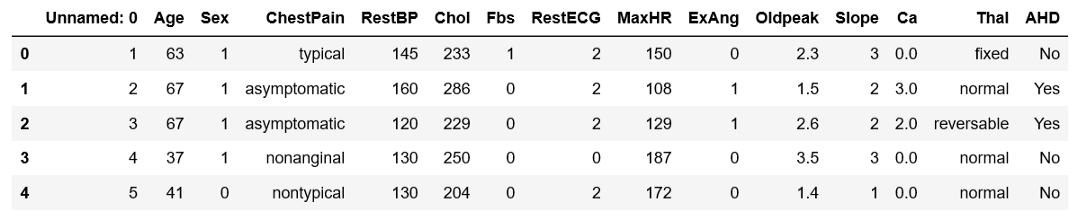The last column of the data is ‘AHD’. It says if a person has heart disease or not. In the beginning, we have a ‘Sex’ column as well. 数据的最后一列是“ AHD”。 它说一个人是否患有心脏病。 一开始，我们也有一个“性别”列。
We are going to construct a CI for the female population proportion that has heart disease. 我们将为患有心脏病的女性人口比例构建一个CI。
First, replace 1 and 0 with ‘Male’ and ‘Female’ in a new column ‘Sex1’. 首先，在新列“ Sex1”中将“ 1”和“ 0”分别替换为“ Male”和“ Female”。
df['Sex1'] = df.Sex.replace({1: "Male", 0: "Female"})We do not need all the columns in the dataset. We will only use the ‘AHD’ column as that contains if a person has heart disease or not and the Sex1 column we just created. Make a DataFrame with only these two columns and drop all the null values. 我们不需要数据集中的所有列。 我们将仅使用“ AHD”列，因为其中包含一个人是否患有心脏病以及我们刚刚创建的Sex1列。 创建一个仅包含这两列的DataFrame并删除所有空值。
dx = df[["AHD", "Sex1"]].dropna()We need the number of females who have heart disease. The line of code below will give the number of males and females with heart disease and with no heart disease. 我们需要患有心脏病的女性人数。 下面的代码行将给出患有心脏病但没有心脏病的男性和女性的数量。
pd.crosstab(dx.AHD, dx.Sex1)Here is the output table: 这是输出表：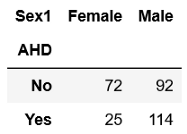The number of females who have heart disease is 25. Calculate the female population proportion with heart disease. 患有心脏病的女性人数为25。 计算患有心脏病的女性人口比例。
p_fm = 25/(72+25)The ‘p_fm’ is 0.26. The size of the female population: 'p_fm'为0.26。 女性人数：
n = 72+25The size of the female population is 97. Calculate the standard error 女性人口的总数为97。 计算标准误
se_female = np.sqrt(p_fm * (1 - p_fm) / n)The standard error is 0.044. 标准误差为0.044。
Now construct the CI using the formulas above. The z-score is 1.96 for a 95% confidence interval. 现在，使用以上公式构造CI 。 对于95％的置信区间，z得分是1.96。
z_score = 1.96lcb = p_fm - z_score* se_female #lower limit of the CIucb = p_fm + z_score* se_female #upper limit of the CIThe confidence interval is 0.17 and 0.344. 置信区间为0.17和0.344。
You can calculate it using the library ‘statsmodels’. 您可以使用“ statsmodels”库进行计算。
import statsmodels.api as smsm.stats.proportion_confint(n * p_fm, n)The confidence interval comes out to be the same as above. 置信区间与上述相同。
演示地址GIPHYGIPHY  人口比例差异的CI (CI for the Difference in Population Proportion)
Is the population proportion of females with heart disease the same as the population proportion of males with heart disease? If they are the same, then the difference in both the population proportions will be zero. 患有心脏病的女性人口比例是否与患有心脏病的男性人口比例相同？ 如果它们相同，则两个人口比例之差将为零。
We will calculate a confidence interval of the difference in the population proportion of females and males with heart disease. 我们将计算患有心脏病的女性和男性人口比例差异的置信区间。
Here is the step by step process: 这是逐步的过程：
Calculate the male population proportion with heart disease and standard error using the same procedure. 使用相同的程序计算患有心脏病和标准误的男性人口比例。
p_male = 114/(114+92)  #male population proportionn = 114+92             #total male populationThe male population proportion with heart disease is 0.55 and the male population size is 206. Calculate the standard error for the male population proportion. 患有心脏病的男性人口比例为0.55，男性人口规模为206。 计算男性人口比例的标准误。
se_male = np.sqrt(p_male * (1 - p_male) / n)The standard error for the male population is 0.034. Calculate the difference in standard error. 男性人群的标准误差是0.034。 计算标准误差的差。
The difference in standard error is not just subtraction. Use proper formula. 标准误差的差异不仅仅是减法。 使用正确的公式。 Here is the formula to calculate the difference in two standard errors: 这是计算两个标准误差之差的公式：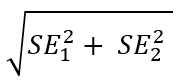Let’s use this formula to calculate the difference in the standard error of male and female population with heart disease. 让我们使用此公式来计算患有心脏病的男性和女性人口的标准误之差。
se_diff = np.sqrt(se_female**2 + se_male**2)Use this standard error to calculate the difference in the population proportion of males and females with heart disease and construct the CI of the difference. 使用此标准误差来计算患有心脏病的男性和女性人口比例的差异，并构建差异的CI。
d = 0.55 - 0.26lcb = d - 1.96 * se_diff  #lower limit of the CIucb = d + 1.96 * se_diff  #upper limit of the CIThe CI is 0.18 and 0.4. This range does not have 0 in it. Both the numbers are above zero. So, We cannot make any conclusion that the population proportion of females with heart disease is the same as the population proportion of males with heart disease. If the CI would be -0.12 and 0.1, we could say that the male and female population proportion with heart disease is the same. CI为0.18和0.4。 此范围内没有0。 两个数字都大于零。 因此，我们无法得出任何结论：患有心脏病的女性人口比例与患有心脏病的男性人口比例相同。 如果CI为-0.12和0.1，则可以说患有心脏病的男性和女性人口比例相同。
演示地址GIPHYGIPHY  均值CI的计算 (Calculation of CI of mean)
We will use the same heart disease dataset. The dataset has a ‘chol’ column that contains the cholesterol level. For this demonstration, 我们将使用相同的心脏病数据集。 数据集有一个“胆固醇”列，其中包含胆固醇水平。 为了这个示范
we will calculate the confidence interval of the mean cholesterol level of the female population. 我们将计算女性人群平均胆固醇水平的置信区间。
Let’s find the mean, standard deviation, and population size for the female population. I want to get the same parameters for the male population as well. Because it will be useful for our next exercise. Use pandas groupby and aggregate methods for this purpose. If you need a refresher on pandas groupby and aggregate method, please check out this article: 让我们找到女性人口的均值，标准差和人口规模。 我也想为男性人口获得相同的参数。 因为它将对我们的下一个练习有用。 为此，请使用pandas groupby和aggregate方法。 如果您需要对pandas groupby和aggregate方法进行复习，请查看本文：
Here is the code to get the mean, standard deviation, and population size of the male and female population: 这是获取男性和女性人口的均值，标准差和人口规模的代码：
df.groupby("Sex1").agg({"Chol": [np.mean, np.std, np.size]})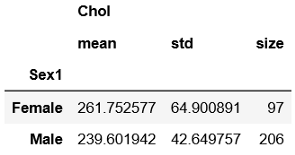If we extract the necessary parameters for the female population only: 如果仅提取女性人口的必要参数：
mean_fe = 261.75  #mean cholesterol of femalesd = 64.9         #standard deviation for female populationn = 97            #Total number of femalez = 1.96          #z-score from the z table mentioned beforeHere 1.96 is the z-score for a 95% confidence level. 这里1.96是95％置信水平的z得分。
Calculate the standard error using the formula for the standard error of the mean 使用公式计算平均值的标准误差
se = sd /np.sqrt(n)Now we have everything to construct a CI for mean cholesterol in the female population. 现在，我们已掌握了构建女性人群平均胆固醇CI的一切方法。
Construct the CI 构建CI
lcb = mean_fe - z* se  #lower limit of the CIucb = mean_fe + z* se  #upper limit of the CI(lcb, ucb)The CI came out to be 248.83 and 274.67. CI分别为248.83和274.67。
That means the true mean of the cholesterol of the female population will fall between 248.83 and 274.67 这意味着女性人群中胆固醇的真实平均值将介于248.83和274.67之间 演示地址GIPHYGIPHY  均值差的CI的计算 (Calculation of CI of The Difference in Mean)
There are two approaches to calculate the CI for the difference in the mean of two populations. 有两种方法可以计算两个总体的均值之差的CI。
Pooled approach and unpooled approach 合并方法和非合并方法 As mentioned earlier, we need a simple random sample and a normal distribution. If the sample is large, a normal distribution is not necessary. 如前所述，我们需要一个简单的随机样本和一个正态分布。 如果样本很大，则不需要正态分布。
There is one more assumption for a pooled approach. That is, the variance of the two populations is the same or almost the same. 合并方法还有一个假设。 也就是说，两个总体的方差相同或几乎相同。 If the variance is not the same, the unpooled approach is more appropriate. 如果方差不相同，则非池化方法更为合适。
The formula of the standard error for the pooled approach is: 合并方法的标准误差公式为：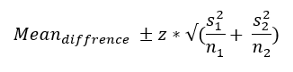Here, s1 and s2 are the standard error for the population1 and population2. In the same way, n1 and n2 are the population size of population1 and population2. s1和s2是总体1和总体2的标准误差。 同样，n1和n2是人口1和人口2的人口规模。
The formula of the standard error for the unpooled approach is: 无池方法的标准误差公式为：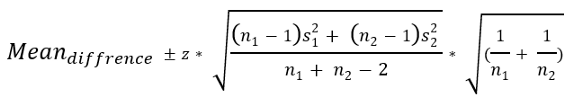Here, we will construct the CI for the difference in mean of the cholesterol level of the male and female population. 在这里，我们将针对男性和女性人群中胆固醇水平平均值的差异构建CI。
We already derived all the necessary parameters from the dataset in the previous example. Here they are: 在前面的示例中，我们已经从数据集中导出了所有必要的参数。 他们来了：
n1 = 97n2 = 206mean_female = 261.75mean_male = 239.6sd_female = 64.9sd_male = 42.65As we can see, the standard deviation of the two target populations is different. So. the variance must be different as well. 我们可以看到，两个目标人群的标准差是不同的。 所以。 方差也必须不同。 So, for this example, the unpooled approach will be more appropriate. 因此，对于此示例，非池化方法将更合适。
Calculate the standard error for male and female population using the formula we used in the previous example 使用上一个示例中使用的公式计算男性和女性人口的标准误差
sem_female = sd_female / np.sqrt(97)sem_male = sd_male / np.sqrt(206)The difference in mean of the two samples 两个样本的均值之差
mean_d = mean_female - mean_maleThe difference in mean ‘mean_d’ is 22.15. 平均值“ mean_d”之差为22.15。
Using the formula for the unpooled approach, calculate the difference in standard error: 使用非池化方法的公式，计算标准误差的差：
sem_d = (np.sqrt((n1-1)*se_female**2 + (n2-1)*se_male**2)/(n1+n2-2))*(np.sqrt(1/n1 + 1/n2))Finally, construct the CI for the difference in mean 最后，针对均值差构造CI
lcb = mean_d - 1.96*sem_d  #lower limit of the CIucb = mean_d + 1.96*sem_d  #upper limit of the CI(lcb, ucb)The lower and upper limit of the confidence interval came out to be 22.1494 and 22.15. They are almost the same. That means the mean cholesterol of the female population is not different than the mean cholesterol of the male population. 置信区间的上限和下限分别为22.1494和22.15。 他们几乎一样。 这意味着女性人口的平均胆固醇与男性人口的平均胆固醇没有差异。
结论 (Conclusion)
In this article, I tried to explain the confidence interval in detail with the calculation process in python. Python code I used here is simple enough for anyone to understand. Even if you are not a python user you should be able to understand the process and apply it in your way. 在本文中，我试图用python中的计算过程详细解释置信区间。 我在这里使用的Python代码非常简单，任何人都可以理解。 即使您不是python用户，您也应该能够理解该过程并以您的方式应用它。
翻译自: https://towardsdatascience.com/a-complete-guide-to-confidence-interval-and-examples-in-python-ff417c5cb593python 置信区间
展开全文• 置信区间是指由 样本统计量 所构造的总体参数的估计区间。这句话也就是说 ( 这里统计量一般指均值 ) 利用样本均值来估计总体均值的可靠程度, 这个"可靠程度"用"置信区间"来表示, 置信区间(是一个系数)取值范围为: 0 ...
置信区间是指由 样本统计量 所构造的总体参数的估计区间。这句话也就是说 ( 这里统计量一般指均值 ) 利用样本均值来估计总体均值的可靠程度, 这个"可靠程度"用"置信区间"来表示, 置信区间(是一个系数)取值范围为: 0 当样本量越大, 则越可靠.置信区间计算公式:ci = mean±stdN(ppf)( (1-α)/2 )公式参数说明:ci: 表示置信区间mean: 表示样本均值std: 表示样本标准差N(ppf): 表示正态分布的百分点函数α : 是显著性水平α的取值跟样本量有关样本量个数α取值0~990.1100~5000.05501~10000.01约20000.001其中;百分点函数ppf是累积分布函数cdf的反函数常用的几个置信区间的计算可以不用上述公式,而使用更简单的公式置信水平是指特定个体对待特定命题真实性相信的程度通常使用 90%，95% 和 99% 作为置信区间的置信水平。这三个置信水平的临界值分别为 1.64，1.98 和 2.32。se是样本标准误, n是样本个数se = mean/pow(n,0.5)置信区间算法:ci = mean-se1.64 置信水平为0.9ci = mean-se1.98 置信水平为0.95ci = mean-se*2.32 置信水平为0.99举例来说，如果在一次大选中某人的支持率为55%，而置信水平(也称为可信区间)0.95以上的置信区间是(50%,60%)，那么他的真实支持率有百分之九十五的机率落在百分之五十和百分之六十之间，因此他的真实支持率不足一半的可能性小于百分之2.5。 如例子中一样，置信水平一般用百分比表示，因此置信水平0.95上的置信空间也可以表达为:95%置信区间。置信区间的两端被称为置信极限。对一个给定情形的估计来说，显著性水平越高，所对应的置信区间就会越大。但是; 重点来了, 在python里可以直接调用函数求出置信区间置信水平 = 可信区间 = 可信度 ;他们是同一个说法(其中可信度是为了方便理解,在案例中我用于替代置信水平的)置信区间宽度 = 置信区间上极限 - 置信区间下极限结论是:置信区间宽度越小,预测模型越精确;但是置信区间宽度小了,可信度也就越小了"""  置信区间  """df = len(data) - 1alpha=    # 是设定的可信区间, 可以理解为可信度; 数值是百分数,取值范围(0,1)ci = stats.t.interval(alpha, df, loc=np.mean(data), scale=stats.sem(data)) # ci是置信区间案例: 对给出的样本数据分析(所用文件是csv格式的,无法导入)import osimport pandas as pdimport matplotlibimport matplotlib.pyplot as pltfrom scipy import statsimport numpy as npos.chdir(r"D:\python金融")data = pd.read_csv("house_price_gr.csv",encoding='gbk') # 文件有中文,encoding= 'gbk'matplotlib.rcParams['axes.unicode_minus']=False#解决保存图像时负号'-'显示为方块的问题plt.rcParams['font.sans-serif'] = ['SimHei'] # 指定默认字体# print(data.shape) # 查看样本数量# 绘制直方图 plt.hist(样本数据,bins=柱子个数) ; 默认x轴是样本数值, y轴是样本数值的个数# plt.hist(data.rate,bins=40)# plt.show()# 查看均值,标准差,百分位数等print(data.rate.describe())alpha = 0.99alpha_list = [] # 可信度列表ci_width_list = [] # 置信区间宽度列表ci_upper_limit = [] # 置信区间上极限ci_lower_limit = [] # 置信区间下极限for i in range(20):print("alpha={}".format(alpha))# 均值的99%可信区间的置信区间"""  置信区间  """df = len(data.rate) - 1ci = stats.t.interval(alpha, df, loc=np.mean(data.rate), scale=stats.sem(data.rate))# ci = stats.t.interval(alpha=0.99,df=len(data.rate)-1,loc=np.mean(data.rate),scale=stats.sem(data.rate))# 算出置信区间的宽度ci_element = cici_lower_limit.append(ci_element)ci_upper_limit.append(ci_element)# ci_element.append(ci)ci_width = ci_element - ci_element # 定义置信区间宽度ci_width_list.append(ci_width)print(ci_width)  # 输出置信区间宽度alpha_list.append(alpha) # 添加到列表中alpha = alpha - 0.05print("置信区间={}".format(ci))print("  "*100)print(ci_width_list)print(alpha_list)x=alpha_list # 设置可信度为x轴# y=ci_width_listplt.plot(x,ci_lower_limit,linestyle="dashdot",label="置信区间下极限")plt.plot(x,ci_width_list,linestyle="dashdot",label = "置信区间宽度")plt.plot(x,ci_upper_limit,linestyle="dashdot",label= "置信区间上极限")plt.xlabel("可信度/百分数") # 可信度指的是可信区间, 为了方面理解,用可信度代替可信区间plt.ylabel("置信区间/单位为变量的单位")plt.title("可信度--置信区间宽度 图")plt.legend() # 显示标签"""可信度与置信区间图说明:例如:可信度=0.90,置信区间宽度=0.05, 置信区间上限=0.07,置信区间下限=0.02则说明:发生在区间(0.02,0.07)这个范围内的可能性为0.90通过图像得出: 置信区间宽度越越小,预测模型越精确;但是置信区间宽度小了,可信度也就越小了"""plt.show()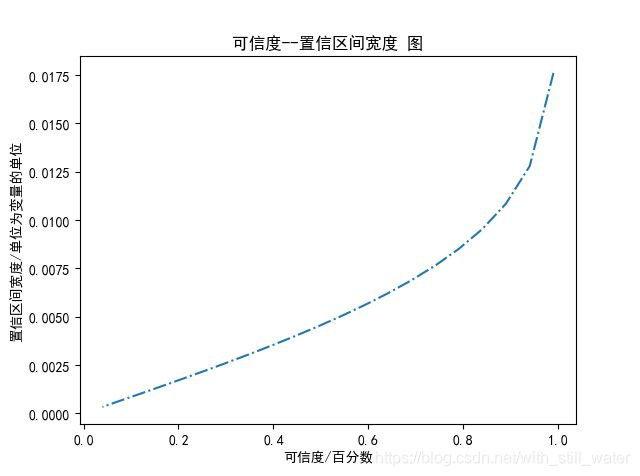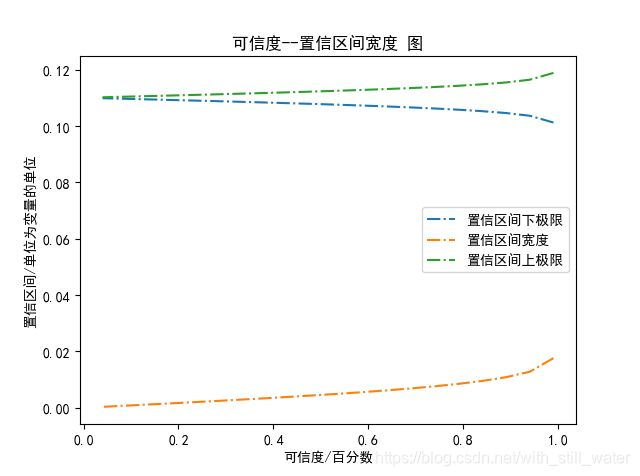通过图可以得出上述结论::置信区间宽度越小,预测模型越精确;但是置信区间宽度小了,可信度也就越小了所有为了得到较高的可信度, 就必须得扩大置信区间宽度; 一般取可信度=0.95来计算
展开全文• python 置信区间置信区间是指由 样本统计量 所构造的总体参数的估计区间。这句话也就是说 ( 这里统计量一般指均值 ) 利用样本均值来估计总体均值的可靠程度, 这个"可靠程度"用"置信区间"来表示, 置信区间(是一个系数...
python 置信区间置信区间是指由 样本统计量 所构造的总体参数的估计区间。这句话也就是说 ( 这里统计量一般指均值 ) 利用样本均值来估计总体均值的可靠程度, 这个"可靠程度"用"置信区间"来表示, 置信区间(是一个系数)取值范围为: 0当样本量越大, 则越可靠.置信区间计算公式:ci = mean±stdN(ppf)( (1-α)/2 )公式参数说明:ci: 表示置信区间mean: 表示样本均值std: 表示样本标准差N(ppf): 表示正态分布的百分点函数α : 是显著性水平α的取值跟样本量有关其中，百分点函数ppf是累积分布函数cdf的反函数常用的几个置信区间的计算可以不用上述公式,而使用更简单的公式置信水平是指特定个体对待特定命题真实性相信的程度通常使用 90%，95% 和 99% 作为置信区间的置信水平。这三个置信水平的临界值分别为 1.64，1.98 和 2.32。se是样本标准误, n是样本个数se = mean/pow(n,0.5)置信区间算法:ci = mean-se1.64 置信水平为0.9ci = mean-se1.98 置信水平为0.95ci = mean-se*2.32 置信水平为0.99举例来说，如果在一次大选中某人的支持率为55%，而置信水平(也称为可信区间)0.95以上的置信区间是(50%,60%)，那么他的真实支持率有百分之九十五的机率落在百分之五十和百分之六十之间，因此他的真实支持率不足一半的可能性小于百分之2.5。 如例子中一样，置信水平一般用百分比表示，因此置信水平0.95上的置信空间也可以表达为:95%置信区间。置信区间的两端被称为置信极限。对一个给定情形的估计来说，显著性水平越高，所对应的置信区间就会越大。但是; 重点来了, 在python里可以直接调用函数求出置信区间置信水平 = 可信区间 = 可信度 ;他们是同一个说法(其中可信度是为了方便理解,在案例中我用于替代置信水平的)置信区间宽度 = 置信区间上极限 - 置信区间下极限结论是:置信区间宽度越小,预测模型越精确;但是置信区间宽度小了,可信度也就越小了"""  置信区间  """df = len(data) - 1alpha=    # 是设定的可信区间, 可以理解为可信度; 数值是百分数,取值范围(0,1)ci = stats.t.interval(alpha, df, loc=np.mean(data), scale=stats.sem(data)) # ci是置信区间案例: 对给出的样本数据分析(所用文件是csv格式的,无法导入)import osimport pandas as pdimport matplotlibimport matplotlib.pyplot as pltfrom scipy import statsimport numpy as npos.chdir(r"D:\python金融")data = pd.read_csv("house_price_gr.csv",encoding='gbk') # 文件有中文,encoding= 'gbk'matplotlib.rcParams['axes.unicode_minus']=False#解决保存图像时负号'-'显示为方块的问题plt.rcParams['font.sans-serif'] = ['SimHei'] # 指定默认字体# print(data.shape) # 查看样本数量# 绘制直方图 plt.hist(样本数据,bins=柱子个数) ; 默认x轴是样本数值, y轴是样本数值的个数# plt.hist(data.rate,bins=40)# plt.show()# 查看均值,标准差,百分位数等print(data.rate.describe())alpha = 0.99alpha_list = [] # 可信度列表ci_width_list = [] # 置信区间宽度列表ci_upper_limit = [] # 置信区间上极限ci_lower_limit = [] # 置信区间下极限for i in range(20):print("alpha={}".format(alpha))# 均值的99%可信区间的置信区间"""  置信区间  """df = len(data.rate) - 1ci = stats.t.interval(alpha, df, loc=np.mean(data.rate), scale=stats.sem(data.rate))# ci = stats.t.interval(alpha=0.99,df=len(data.rate)-1,loc=np.mean(data.rate),scale=stats.sem(data.rate))# 算出置信区间的宽度ci_element = cici_lower_limit.append(ci_element)ci_upper_limit.append(ci_element)# ci_element.append(ci)ci_width = ci_element - ci_element # 定义置信区间宽度ci_width_list.append(ci_width)print(ci_width)  # 输出置信区间宽度alpha_list.append(alpha) # 添加到列表中alpha = alpha - 0.05print("置信区间={}".format(ci))print("  "*100)print(ci_width_list)print(alpha_list)x=alpha_list # 设置可信度为x轴# y=ci_width_listplt.plot(x,ci_lower_limit,linestyle="dashdot",label="置信区间下极限")plt.plot(x,ci_width_list,linestyle="dashdot",label = "置信区间宽度")plt.plot(x,ci_upper_limit,linestyle="dashdot",label= "置信区间上极限")plt.xlabel("可信度/百分数") # 可信度指的是可信区间, 为了方面理解,用可信度代替可信区间plt.ylabel("置信区间/单位为变量的单位")plt.title("可信度--置信区间宽度 图")plt.legend() # 显示标签"""可信度与置信区间图说明:例如:可信度=0.90,置信区间宽度=0.05, 置信区间上限=0.07,置信区间下限=0.02则说明:发生在区间(0.02,0.07)这个范围内的可能性为0.90通过图像得出: 置信区间宽度越越小,预测模型越精确;但是置信区间宽度小了,可信度也就越小了"""plt.show()通过图可以得出上述结论::置信区间宽度越小,预测模型越精确;但是置信区间宽度小了,可信度也就越小了所有为了得到较高的可信度, 就必须得扩大置信区间宽度; 一般取可信度=0.95来计算原文：https://blog.csdn.net/with_still_water/article/details/102511633
展开全文• python 置信区间置信区间是指由 样本统计量 所构造的总体参数的估计区间。这句话也就是说 ( 这里统计量一般指均值 ) 利用样本均值来估计总体均值的可靠程度, 这个"可靠程度"用"置信区间"来表示, 置信区间(是一个系数...
• Python求解正态分布置信区间正态分布和置信区间正态分布(Normal Distribution)又叫高斯分布，是一种非常重要的概率分布。其概率密度函数的数学表达如下：f(x) = \frac{1}{\sqrt{2\pi}\sigma}e^{-\frac{(x - \mu)^2...
• 计算数据的中值95%的置信区间假设有一组数据如下：data = [-0.1, -2.4, -0.1, -0.7, -1.4, -0.9, -3.2, -0.2, -0.3, -0.6, -3.2, -5.5]求中值的置信区间与求数据的均值置信区间的方法是类似的，在这里我不会讨论详细...
• ## Python 计算置信区间

万次阅读 2015-01-16 16:17:02
Python 计算置信区间Python实现http://vassarstats.net/prop1.html 所计算的置信区间 import math #计算置信区间的函数 def calc(r,n): if n print ('r cannot be greater than n.') return if ...
• 这里是shasan代码的缩写版本，计算数组a的平均值的95％置信区间：import numpy as np, scipy.stats as st st.t.interval(0.95, len(a)-1, loc=np.mean(a), scale=st.sem(a))但使用StatsModels的tconfint_mean可以说...
• proc freq data=datain;by group;tables var / missprint nowarn binomial(level=‘1‘ cl=...(对发生的做置信区间，考虑发生数量为0的情况)ods output binomialcls=cl;run;ods output close;datain的格式如下：gr...
• 正态分布和置信区间正态分布(Normal Distribution)又叫高斯分布，是一种非常重要的概率分布。其概率密度函数的数学表达如下：置信区间是对该区间能包含未知参数的可置信的程度的描述。使用SciPy求解置信区间import ...
• bootstrap可以用来估计样本中任何函数(np.mean，st.genextreme.fit，等等)的置信区间，并且有一个Python库：^{}。在以下是作者相关问题question中的数据：import numpy as np, scipy.stats as st, scikits.bootstrap...
• I am trying to calculate the mean and confidence interval(95%) of a column "Force" in a large dataset. I need the result by using the groupby function by grouping different "Classes".When I calculate ...
• How would I calculate the confidence intervals for a LOWESS regression in Python? I would like to add these as a shaded region to the LOESS plot created with the following code (other packages than ...
• 我试图获得一些x,y数据的指数拟合的置信区间(可用here).这是MWE我必须找到最适合数据的指数：from pylab import *from scipy.optimize import curve_fit# Read data.x, y = np.loadtxt('exponential_data.dat', ...
• 文章目录Python求解正态分布置信区间正态分布和置信区间使用SciPy求解置信区间使用Matplotlib绘制正态分布密度曲线正态分布置信区间规律 Python求解正态分布置信区间 正态分布和置信区间 正态分布（Normal ...
• 我的问题涉及到统计学和python，我是这两方面的初学者。我正在运行一个模拟，对于...我不确定我所做的是否正确，也不确定我想做的是否可以做到，但我的问题是如何从曲线拟合产生的协方差矩阵中得到置信区间。代码首...
• 见下文)的数据子集绘制一条功能上任意的非线性回归线(加上置信度和预测区间)。在为自变量x生成的x跨越20个不同的值：x=(20-np.arange(20))**2，并为每个条件复制{}。数据显示x之间的强非线性，如下所示：import ...
• 当我们利用python进行数据清理和筛选时，有时候一串数据的噪点很多，我们需要抽取一部分数据作为样本来进行分析，下面推荐一种基本的数据筛选方法，利用置信区间，例：样本满足正态分布。 置信区间：当给出某个估计...
• 注意 ：获得拟合曲线置信区间的实际答案由Ulrich 在此给出。经过一些研究(见这里 ， 这里和1.96 )，我想出了自己的解决方案。它接受任意X％置信区间并绘制上下曲线。 这是MWE：from pylab import *from scipy....
• 您可以指定绘制轮廓的z值列表.因此,您所要做的就是为您的发行版收集正确的z值.以下是远离峰值的“1,2和3西格玛”的示例：码：import numpy as npimport matplotlib.cm as cmimport matplotlib.mlab as mlabimport ...
• 什么叫【包含置信区间的折线柱状图】？因为图有点复杂，实在不知道应该叫什么名字好。。图片今天导师发来一张图片，就是下面这张，是一篇论文中的插图，他说这张图片画的挺漂亮，想让我用python模仿一下。首先分析...
• 散点图是常用的表现两个变量或多个变量之间相关关系的统计图。它用点的密集程度和趋势来表示变量...此时带置信区间的散点图，不仅可以帮你判断散点趋势还能判断异常值的情况，其绘制步骤与命令如下：sysuse auto,...
• 今天小编就为大家分享一篇Python求解正态分布置信区间教程，具有很好的参考价值，希望对大家有所帮助。一起跟随小编过来看看吧...

# python置信区间python 订阅